| ENGLISH | JAPANESE | \$BEg:j(B \$B=(><(B HIDEAKI SHIMAZAKI, Ph.D.\$B5~ETBg3XBg3X1!>pJs3X8&5f2JCNG=>pJs3X@l96(B \$BFCDj=Z65 Curriculum Vitae (a full CV pdf) \$BM}2=3X8&5f=j(B \$BG>2J3XAm9g8&5f%;%s%?! \$BM}2=3X8&5f=j(B/\$B%^%5%A%e!<%;%C%D9)2JBg3X(B \$BK,Ld8&5f0w(B (2008-2011) \$BF|K\3X=Q?66=2qFCJL8&5f0w(B (2006-2007, 2008-2010) \$B5~ETBg3XM}3X8&5f2J(B \$BJ*M}3X!&1'ChJ*M}3X@l96!!Gn;N(B(\$BM}3X(B) (2007) \$B%8%g%s%:!&%[%W%-%s%9Bg3X0e3XIt?@7P2J3X2J!!=\$;N(B(\$B?@7P2J3X(B) (2003) \$B7DXf5A=NBg3XM}9)3XItJ*M}>pJs9)3X2J!!3X;N(B(\$B9)3X(B) (2000) Address: \$B5~ETBg3X(B \$B5HED%-%c%s%Q%9K\It9=Fb(B \$B;T@n5-G04[(B 201 Email: h.shimazaki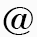i.kyoto-u.ac.jp \$B%R%9%H%0%i%`\$N%S%sI}A*Br(B\$B!C(B\$B%+!<%M%kL)EY?dDj\$N%P%s%II}A*Br(B\$B!C(B\$BF0E*%9%Q%\$%/Aj4X2r@O(B \$B9V5A;qNA(B\$B!C(B\$B%9%Q%\$%/E}7W%b%G%kF~Lg(B\$B!C(B\$B=89g\$B!C(B\$B%5%m%s!&%I!&G>(B\$B!C(B\$B8&5f<<%&%'%V%5%\$%H(B
 \$B!!(B \$B3hF0M=Dj!&Js9p(B \$B!!(B \$B?7Ce>pJs(B 2019\$BG/(B12\$B7n(B7\$BF|!!(B\$B%7%s%.%e%i%j%F%#%5%m%s!JEl5~!K\$G9V1i\$7\$^\$9(B\$B!%(B 2019\$BG/(B11\$B7n(B16\$BF|!!(B\$B%7%s%.%e%i%j%F%#%5%m%s!JBg:e!K\$G9V1i\$7\$^\$9(B\$B!%(B   2019\$BG/(B9\$B7n(B5\$BF|!!(B\$B?@7P2sO)3X2q;o(B9\$B7n9f(B\$B!VCN3P\$N;~4V9=B\$!'G'CN?4M}3X!&?@7P@8M}3X!&7W;;O@\$N;kE@\$+\$i!W(B\$B\$rJT=8\$7\$^\$7\$?!%(B 2019\$BG/(B7\$B7n(B18\$BF|!!(BIJCNN2019\$B\$NH/I=\$G(BJimmy Gaudreault\$B\$5\$s\$,(BBest student award\$B\$r^\$7\$^\$7\$?!%(B 2 student awards in 803 papers from 1532 submissions.   2019\$BG/(B9\$B7n(B17\$BF|!!(BTalk by Miguel Aguilera et al., 15th Granada Seminar on Computational and Statistical Physics. link 2019\$BG/(B9\$B7n(B18\$BF|(B\$B!!(BPoster by Safura Rashid Shomali et al., Bernstein Conference. Berlin, Germany link 2019\$BG/(B9\$B7n(B29\$BF|!!(B\$B>7BT9V1i!!(BThe 7th International Congress on Cognitive Neurodynamics, Italy   2019\$BG/(B8\$B!&(B9\$B7n(B\$B!!@8M}3X8&5f=j\$GFs\$D\$N%\$%Y%s%H\$r3+:E\$7\$^\$7\$?!%(B 8\$B7n(B31\$BF|(B-9\$B7n(B1\$BF|!!(B\$BG>\$N<+M3%(%M%k%.!<86M}%A%e!<%H%j%"%k!&%o!<%/%7%g%C%W(B 9\$B7n(B2\$BF|(B\$B!!@8M}8&8&5f2q(B \$B!V(B\$BG'CN?@7P2J3X\$N@hC<(B \$BG>\$NM}O@\$+\$i?HBN!&@\$3&\$X(B\$B!W(B 2019\$BG/(B8\$B7n(B13\$BF|(B\$B!!>7BT9V1i!!(BData science, Statistics & Visualization (DSSV2019), Kyoto 2019\$BG/(B6\$B7n(B7\$BF|(B \$B!!(B\$B!A%5%m%s!&%I!&G>!A(B\$B\$G9V1i\$7\$^\$7\$?!%(B \$BF|;~!'(B6\$B7n(B7\$BF|!J6b!K(B15:00 - 18:00 \$B>l=j!'5~ETBg3X0e3X8&5f2J(B \$B@hC<2J3X8&5fEo#1(BF \$BBg2q5D<<(B \$B?@7P:YK&=8CD3hF0\$NE}7W2r@O!'G>\$NG.NO3X\$K8~\$1\$F(B \$B;22CEPO?\$O\$3\$A\$i\$+\$i\$*4j\$\$\$^\$9!%!!(Blink 2019\$BG/(B3\$B7n!!(BOIST\$B\$G=8Cf9V5A!&%;%_%J!<\$r\$7\$^\$7\$?!%(Blink\$B!!9V5A;qNA(B 2019\$BG/(B3\$B7n!!(B\$B%7%9%F%`?@7P2J3X%9%W%j%s%0%9%/!<%k\$N1?1D\$K;22C\$7\$^\$7\$?!%(Blink   2018\$BG/(B11\$B7n(B\$B!!(BShomali\$B;a\$NO@J8\$r(BbioRxiv\$B\$K7G:\\$7\$^\$7\$?!%(Blink 2018\$BG/(B7\$B7n!!(B\$BF|K\?@7P2sO)3X2q;o#97n9f!V<+M3%(%M%k%.!<86M}F~Lg!W\$rJT=8\$7\$^\$7\$?!%(Blink 2018\$BG/(B1\$B7n(B\$B!!%K%e!<%i%k%(%s%8%s2>@b\$,(BSpringer\$B\$N=q@R\$N%A%c%W%?!<\$H\$7\$F=PHG\$5\$l\$^\$7\$?!%(Blink   2017\$BG/(B11\$B7n(B14-15\$BF|(B \$B?tM}%b%G%j%s%0%o!<%/%7%g%C%W\$r3+:E\$7\$^\$7\$?!%(Blink 2017\$BG/(B10\$B7n(B5\$BF|(B\$B!!(BLIF\$B%K%e!<%m%s%b%G%k\$N?tM}2r@OO@J8\$,(BJ Computational Neuroscience\$B;o\$K@b\$,(BSpringer\$B\$+\$i=PHG\$5\$l\$kK\\$K7G:\\$5\$l\$^\$9!%(B 2017\$BG/(B9\$B7n(B28\$BF|(B\$B!!6L@nBg3XG>2J3X%o!<%/%7%g%C%W\$G>7BT9V1i\$r9T\$\$\$^\$7\$?!%(B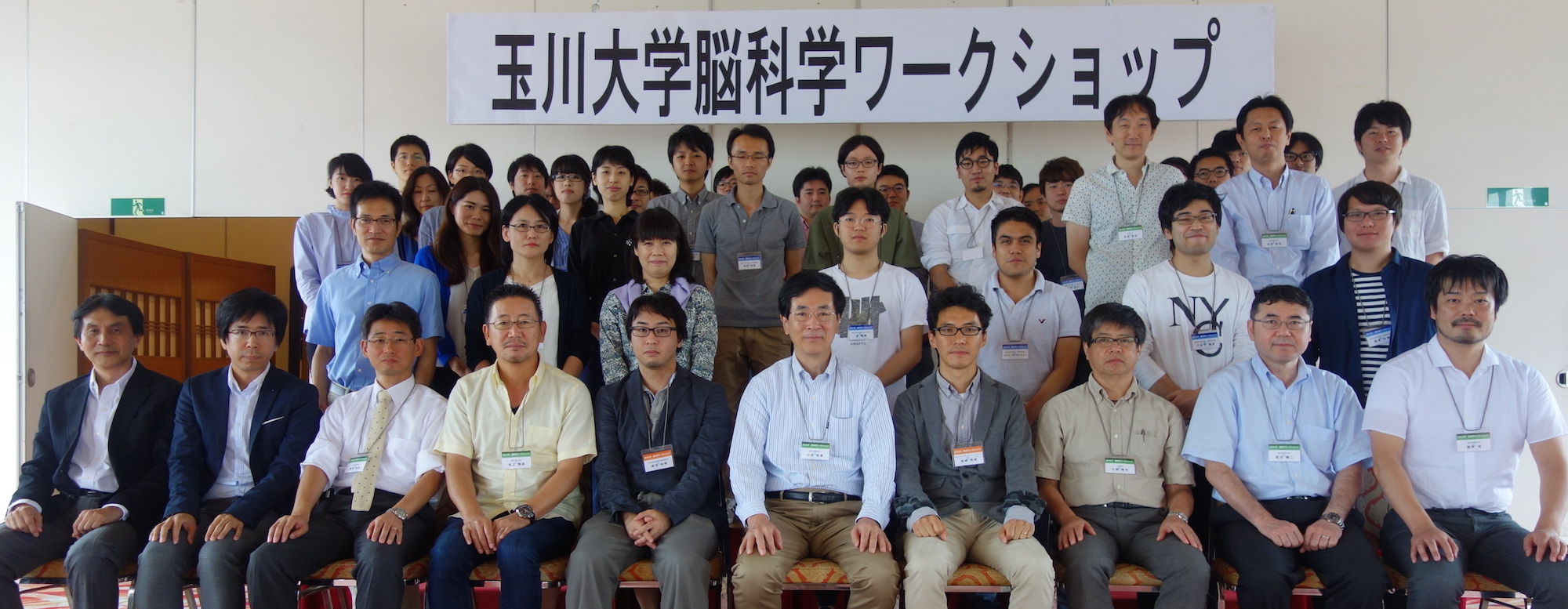2017\$BG/(B8\$B7n(B25\$BF|!!(B\$BF|K\?@7P2sO)3X2q\$N;~8B8&5f2q\$r3+:E\$7\$^\$7\$?!%link] 2017\$BG/(B6\$B7n(B22\$BF|(B\$B!!%_%D%P%ASL3P\$N?tM}%b%G%kO@J8\$,(BPLoS Computational Biology\$B;o\$K7G:\\$5\$l\$^\$7\$?!%(B[link] 2017\$BG/(B4\$B7n(B3\$BF|!!(B\$B5~ETBg3X\$KCeG\$\$7\$^\$7\$?!%6&F18&5f9V:B\$rN)\$A>e\$2\$^\$7\$?!%(B[link] 2017\$BG/(B3\$B7n(B4, 5\$BF|(B\$B!!G>2J3Xlink]2016\$BG/(B12\$B7n(B13\$BF|!!(BDonner, Obermeyer, Shimazaki, Approximate Inference for Time-varying Interactions and Macroscopic Dynamics of Neural Populations \$B\$,(BPLoS Computational Biology\$B\$K 2016\$BG/(B10\$B7n(B20\$BF|(B\$B!!(BChrsitian Donner\$B\$5\$s\$H\$N;E;v\$,(BICONIP2016\$B\$N(BExcellent paper award\$B\$KA*\$P\$l\$^\$7\$?!%(B 2016\$BG/(B5\$B7n(B26\$BF|!!(B\$B6&CxO@J8\$,(BJ Neuroscience\$B;o\$K7G:\\$5\$l\$^\$7\$?(B. [link] 2016\$BG/(B4\$B7n(B1\$BF|(B \$B!!6&CxO@J8\$,%5%\$%(%s%9;o\$K7G:\\$5\$l\$^\$7\$?!%(B[link]   2015\$BG/(B12\$B7n(B24\$BF|(B \$B:G6a\$N9M\$(\$r\$^\$H\$a\$^\$7\$?!%(B Shimazaki H, Neurons as an Information-theoretic Engine. arXiv:1512.07855 [pdf, link] \$BG>Fb\$N>pJsE}9g\$N4pAC\$KMxF@@)8f\$,\$"\$j\$^\$9!%Cm0UEy\$N%U%#!<%I%P%C%/MxF@@)8f\$K\$h\$j463P>pJs\$rJQD4\$9\$k2aDx\$,G.5!4X\$N\$h\$&\$K\$NFbIt7W;;\$NDjNL2=\$d8zN(\$r7W;;\$9\$k\$3\$H\$,\$G\$-\$^\$9!%(B 2015\$BG/(B7\$B7n(B18\$BF|(B\$B!!E}7W?tM}8&5f=j\$G9T\$&8x3+9V:B!V(B\$BE@2aDx%M%C%H%o!<%/\$NE}7W2r@OF~Lg(B\$B!W\$N0FFb\$,\$G\$^\$7\$?!%(B 2015\$BG/(B5\$B7n(B15\$BF|!!(B\$B<+A32hA|2r@O\$NO@J8\$,pdf] 2015\$BG/(B5\$B7n(B\$B!!%+!<%M%kL)EY?dDjO@J8\$,CxL>\$J?@7P2J3XJ Neurophysiol2015. Ito, Zhang, Witter, Moser & Moser Nature 2015. Manita, Suzuki, ..., Larkum & Murayama Neuron 2015. 2015\$BG/(B3\$B7n(B19\$BF|!!(B\$B?@7P:YK&\$N%5%\$%l%s%9\$H9bSimultaneous silence organizes structured higher-order interactions in neural populations. Scientific Reports 5, 9821, 2015. [link]2014\$BG/(B4\$B7nH/Gd(B \$B!V%+%s%G%k?@7P2J3X(B \$BBh#5HG!W(B\$B!J%a%G%#%+%k!&%5%\$%(%s%9!&%\$%s%?!<%J%7%g%J%k\$BM}O@?@7P2J3X!JIUO?(BF\$B!K\$NK]Lu\$rC4Ev\$7\$^\$7\$?!%(B
 \$B9V5A(B \$B9V5A;qNA%Z!<%8\$X(B \$B 2019\$BG/(B12\$B7n(B19\$BF|(B, 2020\$BG/(B1\$B7n(B9\$BF|(B \$B5~BgAm?M(B \$BG>>pJs3X1i=,(B 2019\$BG/(B2\$B7n(B18\$BF|(B\$B!!(B\$BM}2=3X8&5f=jG>2J3X=N(B Statistics for Neuroscience link to a course website \$B2a5n\$N9V5A(B 2019\$BG/(B6\$B7n(B5\$BF|(B, 6\$B7n(B19\$BF|(B\$B!!5~Bg>p(B \$B7W;;O@E*G'CN?@7P2J3X!!3,AX%b%G%k\$N3X=,\$HG>\$N7W;;M}O@(B 2019\$BG/(B1\$B7n(B29\$BF|(B\$B!!(B\$BM}2=3X8&5f=jG>2J3X=N(B Statistics for Neuroscience link to a course website 2018\$BG/(B6\$B7n(B6\$BF|(B, 7\$B7n(B17\$BF|(B\$B!!5~Bg>p(B \$B7W;;O@E*G'CN?@7P2J3X!!3,AX%b%G%k\$N3X=,\$HG>\$N7W;;M}O@(B 2017\$BG/(B11\$B7n(B27\$BF|!!(B\$BM}2=3X8&5f=jG>2J3X=N(B Statistics for Neuroscience link to a course website 2017\$BG/(B10\$B7n(B26\$BF|!\$(B12\$B7n(B21\$BF|!\$(B2019\$BG/(B1\$B7n(B11\$BF|!!(B\$B5~BgAm?M(B \$BG>>pJs3X1i=,!! 2017\$BG/(B6\$B7n(B21\$BF|(B\$B!!5~Bg>p(B \$B7W;;O@E*G'CN?@7P2J3X!!!!(B 2017\$BG/(B3\$B7n(B4, 5\$BF|(B\$B!!(B\$BG>2J3X 2016\$BG/(B12\$B7n(B-3\$B7n!!(B\$B9q:]4pFD65Bg3X(B \$BE}7WJ*M}!!J?EgBg!&Eg:j=(><(B 2016\$BG/(B12\$B7n!!(B\$BM}2=3X8&5f=jG>2J3X=N(B Statistics for Neuroscience I and II \$BEg:j=(>link to a course website 2016\$BG/(B2\$B7n(B10\$BF|!!(B\$BE}7W?tM}8&5f=j8x3+9V:B(B \$BE@2aDx%M%C%H%o!<%/\$NE}7W2r@OF~Lg(B\$B!!>.;3?52p!&Eg:j=(><(B 2015\$BG/(B \$BM}2=3X8&5f=jG>2J3X=N(B Statistics for Neuroscience I and II \$BEg:j=(>link to a course website 2014\$BG/(B12\$B7n(B15\$BF|(B, 22\$BF|(B \$BM}2=3X8&5f=jG>2J3X=N(B Statistics for Neuroscience I and II \$BEg:j=(>link to a course website 2014\$BG/(B5\$B7n(B10\$BF|!!(B\$BL@<#Bg3XBg3X1!M}9)3X8&5f2J(B \$BM}9)3XAm9g9V5A(BD\$BBh#22s(B \$B!VCN3P(B/\$BG'CN\$H?@7P%3!<%G%#%s%08&5fF~Lg!W!V?@7P>pJs\$N2rFI!W(B 2013 Mar 3-12 \$B=8Cf9V5A(B Introduction to statistical models of neural spike train data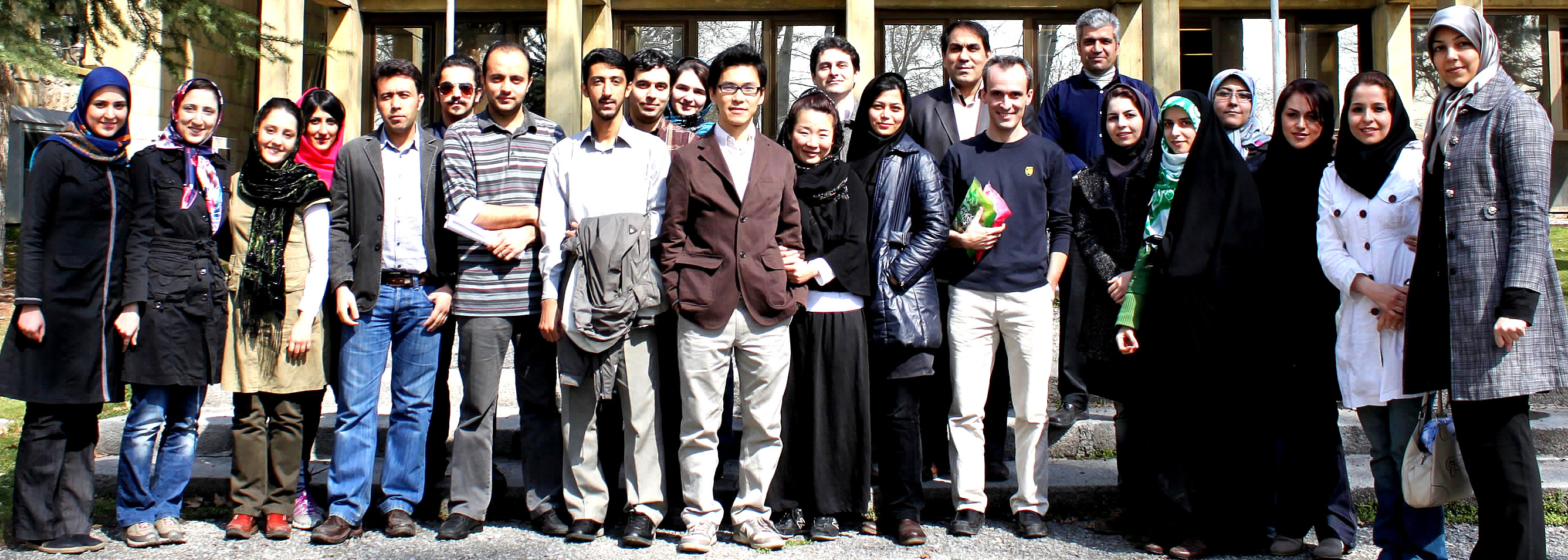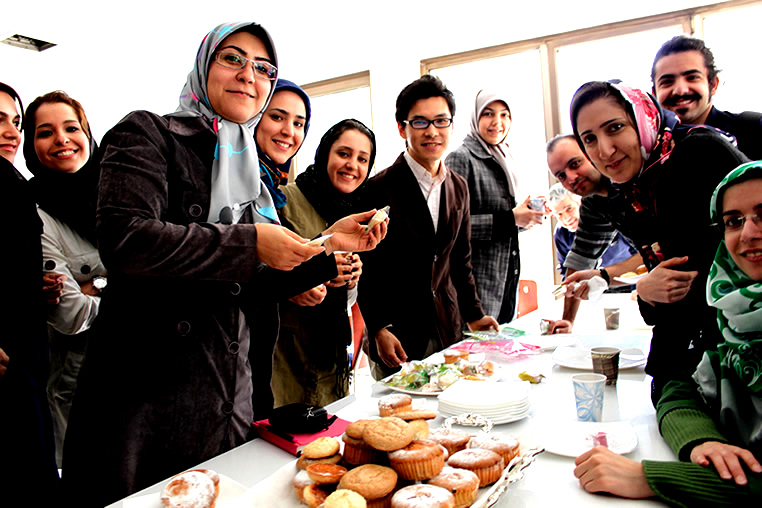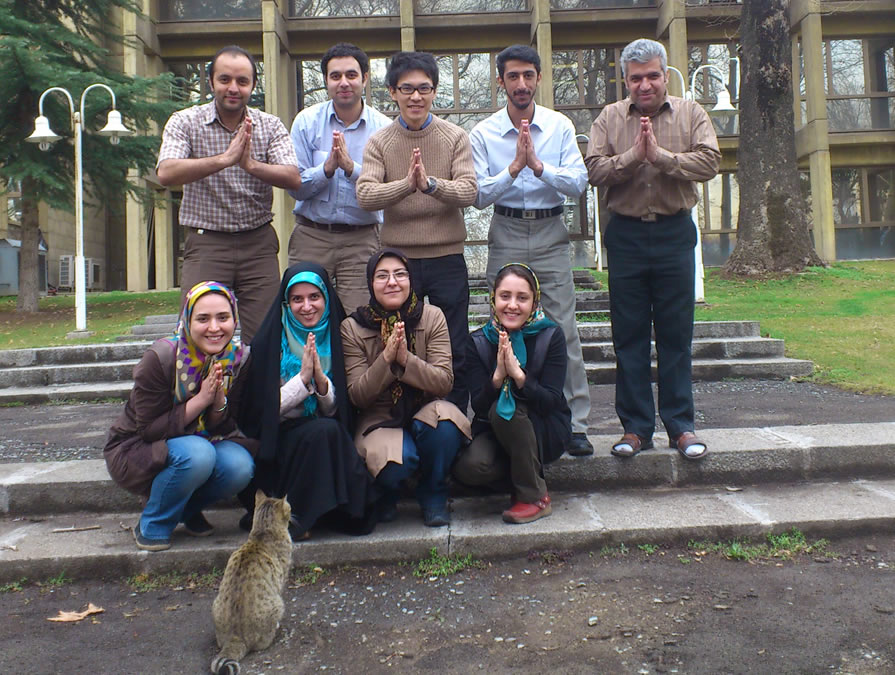The Institute for Research in Fundamental Sciences, School of Cognitive Sciences\$B\$NGn;N2aDx\$NMz=\$2JL\\$H\$7\$FE@2aDx!&0lHL2=@~7A%b%G%k\$rMQ\$\$\$?G>!&?@7P%G!<%?2r@O\$N9V5A!J7W(B6\$B2s!K\$r9T\$\$\$^\$7\$?!%(B
|
\$B8&5fFbMF(B

\$BB?:YK&F1;~5-O??@7P%9%Q%\$%/;~7ONs%G!<%?\$N>uBV6u4V%b%G%k(B

\$B\$,?@7P7O\$N>pJs=hM}\$KK\@b\$,8E\$/\$+\$iDs>'\$5\$l\$F\$\$\$^\$9(B. \$B\$3\$N2>@b\$K=>\$(\$P(B, \$B6(D4E*3hF0\$,J#?t:YK&\$NF14|E*\$JH/2P3hF0\$H\$\$\$&7A\$G8=\$l(B, \$B4QB,\$5\$l\$?%9%Q%\$%/;~7ONs4V\$N(B\$BF14|%9%Q%\$%/(B\$B\$N9=B\$\$K303&;I7c\$dG>\$NFbIt>uBV!JCm0U\$d4|BT!K\$N>pJs\$,Id9f2=\$5\$l\$F\$\$\$k2DG=@-\$,\$"\$j\$^\$9(B. \$B\$3\$&\$7\$?2>@b\$K4p\$E\$/\$BB?:YK&F1;~7WB,5;=Q(B\$B\$NH/E8\$H\$H\$b\$K(B, \$B?@7P:YK&4V\$N%9%Q%\$%/F14|\$,F0J*\$NCN3P!&9TF0\$HL)@\\$J4X78\$K\$"\$k\$3\$H\$r<(:6\$9\$k\$\$\$/\$D\$+\$NZ5r\$,Ds=P\$5\$l\$F\$\$\$^\$9(B.

\$B?@7P:YK&\$NF14|E*6(D43hF0\$rD4\$Y\$k\$?\$a\$K\$O(B, \$BF1;~5-O?\$5\$l\$?J#?t:YK&\$N%9%Q%\$%/;~7ONs4V\$K@x\$`(B\$B%9%Q%\$%/Aj4X9=B\$(B\$B\$r?dDj\$9\$kI,MW\$,\$"\$j\$^\$9(B. \$B\$?\$@\$7(B, \$B#3\$D0J>e\$N?@7P:YK&\$N6(D43hF0\$rD4\$Y\$k\$?\$a\$K\$O#2BN4V\$NAj4X\$@\$1\$G\$J\$/(B, \$B0lHL\$KB?BN4V\$NAj4X(B, \$B\$9\$J\$o\$A(B\$B9b\$B\$b?dDj\$9\$kI,MW\$,\$"\$k\$+\$b\$7\$l\$^\$;\$s(B. \$B\$^\$?6(D43hF0\$,F0E*\$G\$"\$k\$H\$9\$l\$P(B, \$BAj4X9=B\$\$NJQ2=(B\$B\$rDI@W\$G\$-\$J\$1\$l\$P\$J\$j\$^\$;\$s(B. \$B\$3\$&\$7\$?F0E*\$K@8@.!&>C<:(B, \$B\$"\$k\$\$\$O\$=\$NAj4X\$N6/\$5\$,JQ2=\$9\$k2>A[E*\$J6(D4:YK&=8CD\$r(B\$B%;%k%"%;%s%V%j(B\$B\$H8F\$V\$3\$H\$,\$"\$j\$^\$9(B. \$BF1;~5-O?\$7\$??@7P%9%Q%\$%/;~7ONs\$+\$i%;%k%"%;%s%V%j\$rB*\$(\$k\$3\$H\$O2DG=\$J\$N\$G\$7\$g\$&\$+(B.

\$B\$3\$NLdBj\$KEz\$(\$k\$?\$a\$K2f!9\$O(B, \$BBP?t@~7A%b%G%k\$r4QB,%b%G%k\$H\$9\$k(B\$B>uBV6u4V%b%G%k(B\$B\$rMQ\$\$\$F(B, \$B;~4VJQF0\$9\$k%9%Q%\$%/H/2PN(\$H%9%Q%\$%/Aj4X\$rF1;~\$K?dDj\$9\$k5;=Q(B\$B\$r3+H/\$7\$F\$\$\$^\$9(B. \$BBP?t@~7A%b%G%k\$rMQ\$\$\$k\$3\$H\$G(B, \$B9b\$B>pJs4v2?(B\$B\$NOHAH\$_\$K\$h\$j87L)\$KDj5A\$9\$k\$3\$H\$,\$G\$-\$^\$9(B. \$B\$^\$?>uBV6u4V%b%G%k\$O0lHL\$K(B\$B;~4V0MB8%7%9%F%`(B\$B\$N%b%G%k2=\$KMQ\$\$\$i\$l\$kuBV!JBP?t@~7?%b%G%k\$N<+A3Jl?t!K\$N;v8eJ,I[\$rBP?tFs

• \$B86CxO@J8(B
• Donner C., Obermeyer K., Shimazaki H. Approximate Inference for Time-varying Interactions and Macroscopic Dynamics of Neural Populations. PLoS Comp Biol (2017) 13(1): e1005309 [link, code]
• Shimazaki H., Amari S., Brown E. N., and Gruen S., State-space Analysis of Time-varying Higher-order Spike Correlation for Multiple Neural Spike Train Data. PLoS Comput Biol (2012) 8(3): e1002385. doi:10.1371/journal.pcbi.1002385 link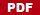• \$B5*MW(B
• Jimmy Gaudreault and Hideaki Shimazaki. State-space analysis of an Ising model reveals contributions of pairwise interactions to sparseness, fluctuation, and stimulus coding of monkey V1 neurons. Lecture Notes in Computer Science (2018) 11141, 641–651
• Donner C. and Shimazaki H. Approximate inference method for dynamic interactions in larger neural populations. ICONIP 2016, Part III, LNCS 9949, 104–110, 2016
• Shimazaki H., Single-trial estimation of stimulus and spike-history effects on time-varying ensemble spiking activity of multiple neurons: a simulation study. J. Phys.: Conf. Ser. (2013) 473 012009 doi:10.1088/1742-6596/473/1/012009 [link]
• Shimazaki H., Amari S., Brown E. N., and Gruen S: State-space Analysis on Time-varying Correlations in Parallel Spike Sequences, Proc. IEEE ICASSP2009, 3501-3504.• \$BEg:j=(><(B, \$B4EMx=S0l(B, Emery Brown, Sonja Gruen. \$BF0E*%9%Q%\$%/Aj4X\$N>uBV6u4V%b%G%k(B. \$BF|K\?@7P2sO)3X2qBh(B19\$B2sA49qBg2q9V1iO@J8=8(B JNNS2009, p.10-11.• \$B2r@bO@J8(B \$B!&5-;v(B
• \$B>7BT9V1i(B
• \$B%]%9%?!
• CNS2009 in Berlin. Shimazaki, Amari, Brown and Gruen. Bayes Factor Analysis for Detection of Time-dependent Higher-order Spike Correlations. [abstract]
• Cosyne2009. Shimazaki, Amari, Brown and Gruen. Detection of non-stationary higher-order spike correlation. [abstract]
• SAND4. Shimazaki, Brown and Gruen: State-space Analysis on Time-dependent Correlation in Parallel Spike Trains. [abstract]
• \$BBh(B11\$B2s>pJsO@E*3X=,M}O@%o!<%/%7%g%C%W(B \$BEg:j=(><(B, \$B%=!<%K%c(B \$B%0%j%e!<%(%s(B: \$BB?:YK&F1;~5-O?%9%Q%\$%/;~7ONs%G!<%?\$N>uBV6u4V%b%G%k!!(B[abstract]
• \$BJsF;(B
• \$B^(B

• \$BC10l?@7P:YK&%9%Q%\$%/;~7ONs%G!<%?\$N%l!<%H?dDj(B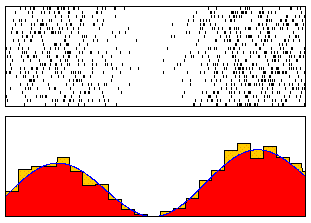\$BEE5\$?@7P@8M}3X\$NF0J*\$BH/2PIQEY!J%9%Q%\$%/%l!<%H!K(B\$B\$NAj4X4X78\$,\$h\$/D4\$Y\$i\$l\$^\$9(B. \$B9-\$/;H\$o\$l\$F\$\$\$k(B\$B%l!<%H?dDj(B\$B\$NPeristimulus Time Histogram (PSTH) \$B\$,\$"\$j\$^\$9(B.

PSTH \$B\$N7A>u\$OJ,3d\$9\$k6h4V\$NI}(B, \$B\$\$\$o\$f\$k(B\$B%S%sI}(B\$B\$KBg\$-\$/0MB8\$9\$k\$K\$b\$+\$+\$o\$i\$:(B, \$BB?\$/\$N>l9g%S%sI}\$O8&5f.\$5\$9\$.\$k\$H%R%9%H%0%i%`\$N%5%s%W%kMI\$i\$.\$,Bg\$-\$/\$J\$j\$^\$9(B. \$B0lJ}\$GBg\$-\$9\$.\$l\$PGX8e\$NJQF0%l!<%H\$K==J,\$KDI=>\$G\$-\$^\$;\$s(B. \$B\$=\$3\$G;d\$?\$A\$O%]%o%=%s2aDx\$H\$\$\$&>r7o2<\$G(B\$B\$B\$rF3\$-\$^\$7\$?(B. \$B\$^\$?=jK>\$N%S%sI}\$rF@\$k\$N\$KI,MW\$J

\$B\$3\$N\$[\$+\$K(B, \$B%+!<%M%k4X?t(B\$B\$rMQ\$\$\$?%l!<%H?dDj\$N\$?\$a\$N(B\$B%+!<%M%k%P%s%II}:GE,2=\$N8x<0(B[4,5,6], \$B\$5\$i\$K?J\$s\$@%H%T%C%/\$H\$7\$F:GE,I}\$,6I=j%G!<%?\$KE,1~\$7\$FJQF0\$9\$k(B\$B2DJQI}%+!<%M%kK!(B\$B\$N8&5f(B[4,6]\$B\$b9T\$C\$F\$\$\$^\$9(B.

\$B%R%9%H%0%i%`:GE,2=(B\$B!!(B

 The optimal bin width of a histogram is obtained as a minimizer of the formula,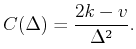Here, k and v are mean and variance of the number of samples in the bins. [Web\$B%"%W%j%1!<%7%g%s(B]

\$B%+!<%M%k:GE,2=(B\$B!!(B

 The optimal bandwidthis obtained as a minimizer of the formula,Here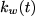andare a kernel function with bandwidthand the data. [Web\$B%"%W%j%1!<%7%g%s(B]